How to Render 3D Surfaces in PHP using 2D Contour Plots - PHP Contour Plot package blog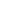All package blogs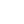PHP Contour PlotPHP Contour Plot package blog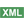How to Render 3D Surf...Post a comment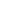See comments (0) Trackbacks (0)

Author:

Viewers: 405

Last month viewers: 11

Package: PHP Contour Plot

Contour plots are graphics that render lines that connect points in a geographic region that have the same value, for instance points in a terrain with the same altitude. They are useful for instance to represent 3D features of a terrain in a 2D image.By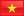tetramatrix.github.io/bbrb...

<email contact>

Introduction

Some applications require the visualization of 3 dimensional surfaces in 2 dimensional image.

Contour plots allow us to render a representation of 3 dimensional surfaces that represent irregular shapes like for instance from a geographic map.3 Dimensional Surfaces

One example that can be rendered as a contour plot  is one that represents the height of the terrain in geographic maps. Another example is the representation of temperature in weather maps.

This kind of graph or diagram is especially useful for agriculture (wheather forecasts), tourism, renewable energy, etc..

Consider this, for each value that you need to represent on these maps, you need 3 values: X value represents the distance on x-axis, Y value represents distance the y-value, and the Z value represents a magnitude value that we would view in the z-axis if the diagram was in 3 dimensions, but we need to represent it somehow in 2 dimensions.

The PHP Contour Plot package solves the problem by plotting lines that have a constant Z value. These lines are called contours when represented in a 2 dimensional diagram. That is, given a value for z, lines are drawn for connecting the (x,y) coordinates where the same Z value occurs.

The simplest tiled map rendering solutions use the Marching Square algorithm. This package uses the Delaunay Triangulation to tile a map. Specifically it uses the Incremental Delaunay Triangulation which is very fast and also very easy to implement.

A Delaunay Triangulation is more accurate then a squared map but also more difficult to understand. A more advance algorithm is Kriging.

When the Delaunay Triangulation is computed (it is time consuming because it users very difficult math) the Z value is mapped along each edge of the triangulation. For instance it uses the statewide average z value  (approximately max(z)+min(z))/2) and interpolates the z slices/values from the z value of the triangulation.

Shapefile and Corners

Another problem is that a geographic map is not a convex hull but there are corners of the map that points towards the interior, i.e. the center of the map. In particular it does not look nice when this corner intersects with the triangulation and creates multiple polygons.

A shapefile is usually a text file with the coordinates of all points that have the same Z value.

To address this issue, this package creates a virtual bitmap of the shapefile and uses a fast pixel-per-pixel hit-test if there is a corner that intersects with a triangulation. Other solutions do not perfectly match the borders of the shapefile and/or are very slow to compute.

If the pixel of the triangulation intersects or overlaps the shapefile it then gets simply deleted. The algorithm is very fast because it needs only to test for  the color of the virtual bitmap. It can use the PHP functions imagecolorat() and imagesetpixel().

A geographic shape is often very irregular due to concave corners and isolated shapes (islands). Therefore the algorithm also computes a minimum bounding box from the shapefile to minimize the hit-test.

PHP Contour plot uses 2 colors and grey shades between the 2 colors to represent the difference in the z value compared to the statewide average z value. Specifically it lets you define a constant (STEPS) to perform linear interpolation to transform the colors to a smoother visualization.

In the generated image, a color is represented by red, green and blue (RGB). The other colors and especially the shades of a color use the greyshade of a color. The greyshade level is other parameter of the color RGB value.

Interpolation

To create a linear interpolation of the values, this package finds the biggest z value and compute the difference (delta z) of the biggest z value and the statewide average z value and maps it to a range of values between 0 and 255. That is the minimum and maximum value for the RGB color representation. Then the resulting RGB value is used in the the graph diagram.

Basic Example of the PHP Contour Plot Package

The PHP Contour Plot package requires PHP 5.3 or later. It can be downloaded from the package page here in the PHP Classes site.

require_once( "contour.php" );

define("ALPHA", 12.5);
define("STEPS", 6);

\$plot = new Contourplot();
\$res = \$plot->main( \$D, \$x_size, \$y_size, \$A, \$mean, ALPHA);
\$pic = new Image( \$plot );
\$pic->draw( \$im );

ImageGIF(\$im);
ImageDestroy(\$im);

A More In Depth Tutorial

The generation of a a contour plot creation is divided into 2 parts: the algorithm and the graphical presentation.

The PHP Contour Plot package expects two constants to be defined with the names ALPHA and STEPS.

The ALPHA Constant

The ALPHA value is a positive number from 0 to infinity. It is used to hide or delete edges longer then the average of the statewide euclidian distance (alpha shape).

A value of 1 means that if the edges are greater or equal, then the average get deleted. A value above 1 means edges greater or equal then the average multiplied the ALPHA value get deleted. A value below 1 means edges greater or equal then the average multiply by ALPHA get deleted.

For instance you can set ALPHA to 12.5:

define("ALPHA" ,12.5);

The STEPS Constant

The STEPS value lets you define how the 3 dimensional surface is plotted in the 2 dimensional image. The value is a positive number and the higher the value the better it can be distinction between the statewide average of the z-value and the minimum and maximum of the z-value.

For instance you set set STEPS to 6:

define("STEPS", 6);

When you have define the constants include the file contour.php in your script, for instance using:

require_once("contour.php");

Creation and Computation

To create a contour plot create a new object of the Contourplot class:

\$plot=new Contourplot();

Then call the object main function. The main function takes 6 arguments:

\$D is the 3 dimensional surface
\$x_size is the width of image
\$y_size is the height of the image
\$A is the geographic shapefile
\$mean is the statewide average of the z-value
ALPHA is a constant Alphashape
\$res = \$plot->main( \$D, \$x_size, \$y_size, \$A, \$mean, ALPHA);
The main function returns the number of triangles. The class also creates internal data from the input like the indices of the triangles (in object->indices), the triangles (in object->delaunay) and the convex hull (in object->hull).

Rendering the Graph

The second part of the work of the class is to implement the algorithm to draw the contour plot diagram.

Create a new object Image from the graph class and feed it with an imagemagick resource. Use the function draw() to render the image and output it to show in the browser.

\$pic = new Image(\$plot);
\$pic->draw(\$im);
ImageGIF(\$im);
ImageDestroy(\$im);

Conclusion

Contour plots can be useful for many applications that need to generate visualizations of how certain physical measurements like altitude or temperature vary within a given geographic region.

Despite they requires some relatively complex algorithms, the PHP Contour package makes it easy to generate this kind of diagrams in your PHP applications.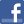Facebook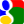Gmail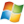Hotmail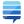StackOverflow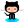GitHub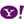YahooPost a commentSee comments (0) Trackbacks (0)All package blogsPHP Contour PlotPHP Contour Plot package blogHow to Render 3D Surf...Advertise on this siteSite mapNewsletterStatisticsSite tipsPrivacy policyContact